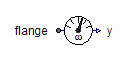Logarithm - MapleSim Help

Logarithm

Compute the natural logarithm or compute the logarithm using a specified baseDescription The Logarithm (or Log) component computes the element-wise natural logarithm (base $ⅇ$) of the input $u$. If base is specified to be something other than $ⅇ$, then it computes ${\mathrm{log}}_{\mathrm{base}}\left(u\right)$. Each input must be greater than 0. The Signal Size parameter assigns the dimension of the input and output connectors.
 Equations ${y}_{j}=\mathrm{log}\left({u}_{j}\right)$

Connections

 Name Description Modelica ID $u$ Real input vector u $y$ Real output vector y

Parameters

 Name Default Units Description Modelica ID Signal Size $1$ Dimension of input and output signals signalSize base $\mathrm{Modelica}\cdot \mathrm{Constants}\cdot e$ Base of logarithm base

 Modelica Standard Library The component described in this topic is from the Modelica Standard Library. To view the original documentation, which includes author and copyright information, click here.